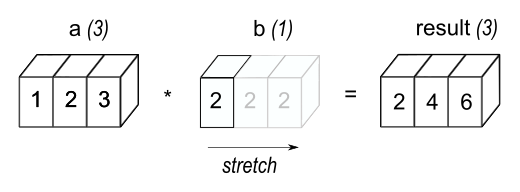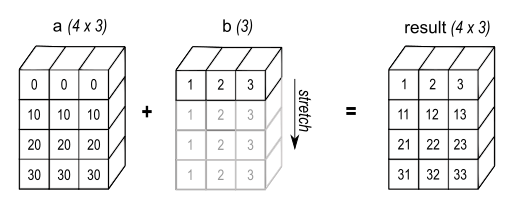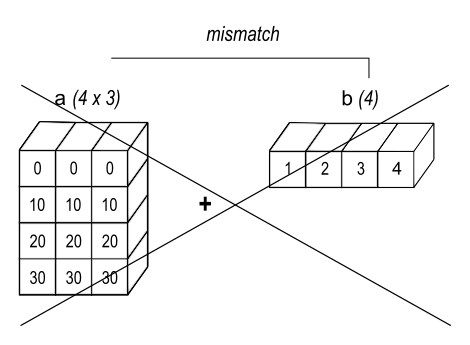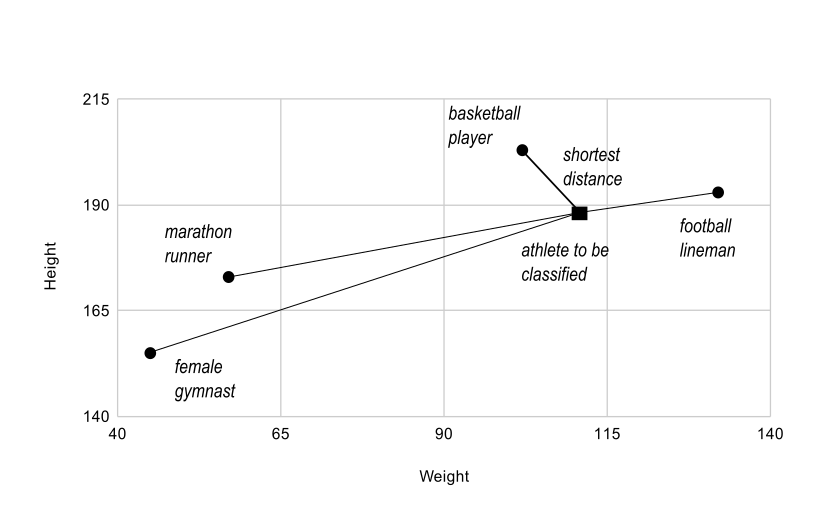The term broadcasting describes how NumPy treats arrays with different shapes during arithmetic operations. Subject to certain constraints, the smaller array is “broadcast” across the larger array so that they have compatible shapes. Broadcasting provides a means of vectorizing array operations so that looping occurs in C instead of Python. It does this without making needless copies of data and usually leads to efficient algorithm implementations. There are, however, cases where broadcasting is a bad idea because it leads to inefficient use of memory that slows computation.

NumPy operations are usually done on pairs of arrays on an element-by-element basis. In the simplest case, the two arrays must have exactly the same shape, as in the following example:

```>>> a = np.array([1.0, 2.0, 3.0])
>>> b = np.array([2.0, 2.0, 2.0])
>>> a * b
array([2.,  4.,  6.])
```

NumPy’s broadcasting rule relaxes this constraint when the arrays’ shapes meet certain constraints. The simplest broadcasting example occurs when an array and a scalar value are combined in an operation:

```>>> a = np.array([1.0, 2.0, 3.0])
>>> b = 2.0
>>> a * b
array([2.,  4.,  6.])
```

The result is equivalent to the previous example where `b` was an array. We can think of the scalar `b` being stretched during the arithmetic operation into an array with the same shape as `a`. The new elements in `b`, as shown in Figure 1, are simply copies of the original scalar. The stretching analogy is only conceptual. NumPy is smart enough to use the original scalar value without actually making copies so that broadcasting operations are as memory and computationally efficient as possible.Figure 1# In the simplest example of broadcasting, the scalar b is stretched to become an array of same shape as a so the shapes are compatible for element-by-element multiplication.

The code in the second example is more efficient than that in the first because broadcasting moves less memory around during the multiplication (`b` is a scalar rather than an array).

When operating on two arrays, NumPy compares their shapes element-wise. It starts with the trailing (i.e. rightmost) dimension and works its way left. Two dimensions are compatible when

1. they are equal, or

2. one of them is 1.

If these conditions are not met, a `ValueError: operands could not be broadcast together` exception is thrown, indicating that the arrays have incompatible shapes.

Input arrays do not need to have the same number of dimensions. The resulting array will have the same number of dimensions as the input array with the greatest number of dimensions, where the size of each dimension is the largest size of the corresponding dimension among the input arrays. Note that missing dimensions are assumed to have size one.

For example, if you have a `256x256x3` array of RGB values, and you want to scale each color in the image by a different value, you can multiply the image by a one-dimensional array with 3 values. Lining up the sizes of the trailing axes of these arrays according to the broadcast rules, shows that they are compatible:

```Image  (3d array): 256 x 256 x 3
Scale  (1d array):             3
Result (3d array): 256 x 256 x 3
```

When either of the dimensions compared is one, the other is used. In other words, dimensions with size 1 are stretched or “copied” to match the other.

In the following example, both the `A` and `B` arrays have axes with length one that are expanded to a larger size during the broadcast operation:

```A      (4d array):  8 x 1 x 6 x 1
B      (3d array):      7 x 1 x 5
Result (4d array):  8 x 7 x 6 x 5
```

A set of arrays is called “broadcastable” to the same shape if the above rules produce a valid result.

For example, if `a.shape` is (5,1), `b.shape` is (1,6), `c.shape` is (6,) and `d.shape` is () so that d is a scalar, then a, b, c, and d are all broadcastable to dimension (5,6); and

• a acts like a (5,6) array where `a[:,0]` is broadcast to the other columns,

• b acts like a (5,6) array where `b[0,:]` is broadcast to the other rows,

• c acts like a (1,6) array and therefore like a (5,6) array where `c[:]` is broadcast to every row, and finally,

• d acts like a (5,6) array where the single value is repeated.

Here are some more examples:

```A      (2d array):  5 x 4
B      (1d array):      1
Result (2d array):  5 x 4

A      (2d array):  5 x 4
B      (1d array):      4
Result (2d array):  5 x 4

A      (3d array):  15 x 3 x 5
B      (3d array):  15 x 1 x 5
Result (3d array):  15 x 3 x 5

A      (3d array):  15 x 3 x 5
B      (2d array):       3 x 5
Result (3d array):  15 x 3 x 5

A      (3d array):  15 x 3 x 5
B      (2d array):       3 x 1
Result (3d array):  15 x 3 x 5
```

Here are examples of shapes that do not broadcast:

```A      (1d array):  3
B      (1d array):  4 # trailing dimensions do not match

A      (2d array):      2 x 1
B      (3d array):  8 x 4 x 3 # second from last dimensions mismatched
```

An example of broadcasting when a 1-d array is added to a 2-d array:

```>>> a = np.array([[ 0.0,  0.0,  0.0],
...               [10.0, 10.0, 10.0],
...               [20.0, 20.0, 20.0],
...               [30.0, 30.0, 30.0]])
>>> b = np.array([1.0, 2.0, 3.0])
>>> a + b
array([[  1.,   2.,   3.],
[11.,  12.,  13.],
[21.,  22.,  23.],
[31.,  32.,  33.]])
>>> b = np.array([1.0, 2.0, 3.0, 4.0])
>>> a + b
Traceback (most recent call last):
ValueError: operands could not be broadcast together with shapes (4,3) (4,)
```

As shown in Figure 2, `b` is added to each row of `a`. In Figure 3, an exception is raised because of the incompatible shapes.Figure 2# A one dimensional array added to a two dimensional array results in broadcasting if number of 1-d array elements matches the number of 2-d array columns.Figure 3# When the trailing dimensions of the arrays are unequal, broadcasting fails because it is impossible to align the values in the rows of the 1st array with the elements of the 2nd arrays for element-by-element addition.

Broadcasting provides a convenient way of taking the outer product (or any other outer operation) of two arrays. The following example shows an outer addition operation of two 1-d arrays:

```>>> a = np.array([0.0, 10.0, 20.0, 30.0])
>>> b = np.array([1.0, 2.0, 3.0])
>>> a[:, np.newaxis] + b
array([[ 1.,   2.,   3.],
[11.,  12.,  13.],
[21.,  22.,  23.],
[31.,  32.,  33.]])
```

Here the `newaxis` index operator inserts a new axis into `a`, making it a two-dimensional `4x1` array. Combining the `4x1` array with `b`, which has shape `(3,)`, yields a `4x3` array.

## A Practical Example: Vector Quantization#

Broadcasting comes up quite often in real world problems. A typical example occurs in the vector quantization (VQ) algorithm used in information theory, classification, and other related areas. The basic operation in VQ finds the closest point in a set of points, called `codes` in VQ jargon, to a given point, called the `observation`. In the very simple, two-dimensional case shown below, the values in `observation` describe the weight and height of an athlete to be classified. The `codes` represent different classes of athletes.  Finding the closest point requires calculating the distance between observation and each of the codes. The shortest distance provides the best match. In this example, `codes` is the closest class indicating that the athlete is likely a basketball player.

```>>> from numpy import array, argmin, sqrt, sum
>>> observation = array([111.0, 188.0])
>>> codes = array([[102.0, 203.0],
...                [132.0, 193.0],
...                [45.0, 155.0],
...                [57.0, 173.0]])
>>> diff = codes - observation    # the broadcast happens here
>>> dist = sqrt(sum(diff**2,axis=-1))
>>> argmin(dist)
0
```

In this example, the `observation` array is stretched to match the shape of the `codes` array:

```Observation      (1d array):      2
Codes            (2d array):  4 x 2
Diff             (2d array):  4 x 2
```Figure 5# The basic operation of vector quantization calculates the distance between an object to be classified, the dark square, and multiple known codes, the gray circles. In this simple case, the codes represent individual classes. More complex cases use multiple codes per class.

Typically, a large number of `observations`, perhaps read from a database, are compared to a set of `codes`. Consider this scenario:

```Observation      (2d array):      10 x 3
Codes            (3d array):   5 x 1 x 3
Diff             (3d array):  5 x 10 x 3
```

The three-dimensional array, `diff`, is a consequence of broadcasting, not a necessity for the calculation. Large data sets will generate a large intermediate array that is computationally inefficient. Instead, if each observation is calculated individually using a Python loop around the code in the two-dimensional example above, a much smaller array is used.

Broadcasting is a powerful tool for writing short and usually intuitive code that does its computations very efficiently in C. However, there are cases when broadcasting uses unnecessarily large amounts of memory for a particular algorithm. In these cases, it is better to write the algorithm’s outer loop in Python. This may also produce more readable code, as algorithms that use broadcasting tend to become more difficult to interpret as the number of dimensions in the broadcast increases.

Footnotes